# NCERT Solutions for Class 7 Maths Chapter 6: Triangle and Its Properties

## NCERT Solutions for Class 7 Maths Chapter 6

In this page we have NCERT Solutions for Class 7 Maths Chapter 6: Triangle and Its Properties All Exercises 6.1,6.2,6.3,6.4,6.5 . Hope you like them and do not forget to like , social share and comment at the end of the page.
Topics
• The line segment joining a vertex of a triangle to the mid point of its opposite side is called a median of the triangle. A triangle has 3 medians.
• The perpendicular line segment from a vertex of a triangle to its opposite side is called an altitude of the triangle. A triangle has 3 altitudes.
• An exterior angle of a triangle is formed, when a side of a triangle is produced. At each vertex, you have two ways of forming an exterior angle.
• The measure of any exterior angle of a triangle is equal to the sum of the measures of its interior opposite angles.
• The total measure of the three angles of a triangle is 180°
• The sum of the lengths of any two sides of a triangle is greater than the length of the third side (a +b > c)
• The difference between the lengths of any two sides is smaller than the length of the third side.
• In a right-angled triangle, the square on the hypotenuse = the sum of the squares on its legs.

### Exercise 6.1

Question 1
In Δ PQR, D is the mid-point of QR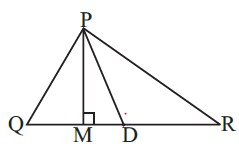PM is _________________.
PD is _________________.
Is QM = MR?
Solution
(i) Altitude
(ii) Median
(iii) No
Question 2.
Draw rough sketches for the following:
(a) In ΔABC, BE is a median.
(b) In ΔPQR, PQ and PR are altitudes of the triangle.
(c) In ΔXYZ, YL is an altitude in the exterior of the triangle.
Solution
(a)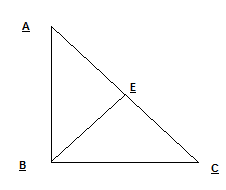(b)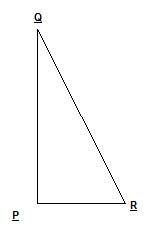(c)Question 3
Verify by drawing a diagram if the median and altitude of an isosceles triangle can be same.
Solution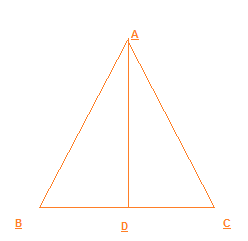AD is the altitude in the Isosceles triangle and we can see that BD=DC, So it is also the median of the triangle

## Try these questions from NCERT book

1. Write the six elements (i.e., the 3 sides and the 3 angles) of $\Delta ABC$.
3 Sides : AB,BC,AC
3 Angles: $\angle ABC, \angle BAC,\angle ACB$

2. Write the:
(i) Side opposite to the vertex Q of $\Delta PQR$
(ii) Angle opposite to the side LM of $\Delta LMN$
(iii) Vertex opposite to the side RT of $\Delta RST$
a. PR
b.$\angle LNM$
c. S

3. Look at Fig 6.2 and classify each of the triangles according to its
(a) Sides
(b) Angles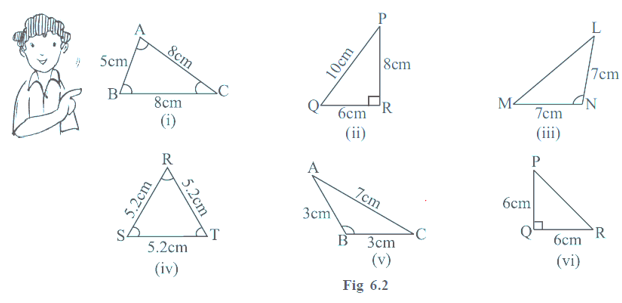Based on Sides
i. Isosceles
ii.Scalene
iii. Isosceles
iv. Equilateral
v. Isosceles
vi. Isosceles
Based on angles
i.Acute-angled
ii. Right-angled
iii.Obtuse-angled
iv.Acute-angled
v. Obtuse-angled
vi.Right-angled

4. How many medians can a triangle have?
3

5.Does a median lie wholly in the interior of the triangle? (If you think that this is not true, draw a figure to show such a case).
Yes it always lie in the interior of the triangle

6. How many altitudes can a triangle have?
3

7. Will an altitude always lie in the interior of a triangle? If you think that this need not be true, draw a rough sketch to show such a case.
No it can be outside

8. Can you think of a triangle in which two altitudes of the triangle are two of its sides?
Yes ,it is the case with right angle triangled

9. Can the altitude and median be same for a triangle?
Yes it can be same in case equilateral tringle

### Exercise 6.2

Question 1
Find the value of the unknown exterior angle in the below figures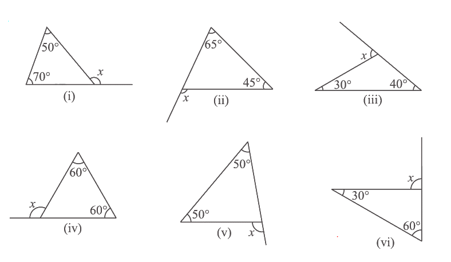Solution
We know that an exterior angle of a triangle is equal to the sum of its interior opposite
angles.
(i) $x=50+70 =120^0$
(ii) $x=45+65 =110^0$
(iii) $x= 30+40=70^0$
(iv) $x= 60+60 =120^0$
(v) $x=50+50 =100^0$
(vi) $x=30+60=90^0$
Question 2
Find the value of the unknown interior angle x in the following figures: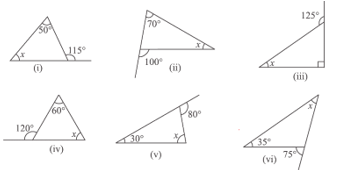Solution
(i) $115= x+50$
$x=115-50=65^0$
(ii) $100 + x=70$
$x=100-70=30^0$
(iii) $x+90=125$
$x=35^0$
(iv) $x+60=120$
$x=60^0$
(v) $x+30=80$
$x=50^0$
(vi) $x+35=75$
$x=40^0$

## Try these questions

1. An exterior angle of a triangle is of measure 70° and one of its interior opposite angles is of measure 25°. Find the measure of the other interior opposite angle.
We know that
Sum of interior opposite angles = Exterior angle
then
x+ 25=70
x=45°

2. The two interior opposite angles of an exterior angle of a triangle are 60° and 80° . Find the measure of the exterior angle.
We know that
Sum of interior opposite angles = Exterior angle
then
Exterior angle=60 + 80 =140deg;

3. Is something wrong in this diagram (Fig 6.12)? Comment.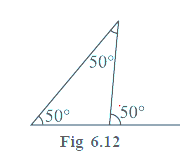We know that
Sum of interior opposite angles = Exterior angle
Here
50=50+50.
Which is not true.So these angles are not possible in triangle

### Exercise 6.3

Question 1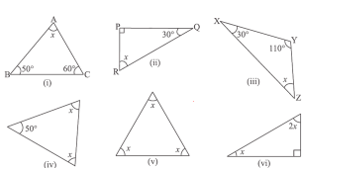Find the value of the unknown x in the following diagrams:
Solution
The sum of all interior angles of a triangle is 180°. By using this property, these problems can be solved as follows.
(i) x + 50° + 60° = 180°
x + 110° = 180°
x = 180° -110° = 70°
(ii) x + 90° + 30° = 180°
x +120° = 180°
x = 180°- 120° = 60°
(iii) x+30° +110° = 180°
x+ 140° = 180°
x = 180°- 140° = 40°
(iv) 50° + x + x = 180°
50° +2x = 180°
2x = 180°- 50° = 130°
x=650
(v) x + x + x = 180°
3x = 180°
x=600
(vi) x + 2x + 90° = 180°
3x = 180° – 90° = 900
x=300

Question 2
Find the values of the unknown’s x and y in the following diagrams: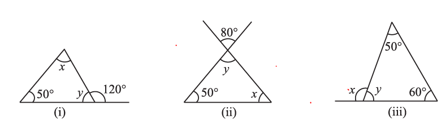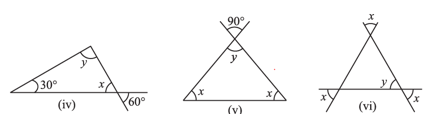Solution:
(i) y + 120° = 180° (Linear pair)
y = 180° — 1200 = 600
x+ y + 50° = 180° (Angle sum property)
x+ 60° + 50° = 180°
x=700
(ii) y = 80° (Vertically opposite angles)
y + x + 50° = 180° (Angle sum property)
80° + x + 50° = 180°
x+ 1300 = 180°
x=500
(iii) y + 50° + 60° = 180° (Angle sum property}
y = 180° — 60° – 50° = 700
x+ y = 180 (Linear pair)
x= 180° — y = 180° — 70° = 110°
iv) x=600 ( Vertically opposite angles)
Now
x+y+30 =180
60+y+30=180
y=90
v) x=900 ( Vertically opposite angles)
Now,
x+x+y=180
2x+90=180
X=450
vi)
y=x ( Vertically opposite angles)
Other two angles are also angle x as vertically opposite angles
y+x+x=180
x+x+x=180
3x=180
x=600

1. Two angles of a triangle are 30° and 80°. Find the third angle.
$x+ 30 +80=180$
x=70°

2. One of the angles of a triangle is 80° and the other two angles are equal. Find the measure of each of the equal angles.
$x+x+80=180$
x=50°

3. The three angles of a triangle are in the ratio 1:2:1. Find all the angles of the triangle.
$x + 2x + x=180$
x=45
So angles are 45°,90°,45°

4. Can you have a triangle with two right angles?
False as then Angle sum property will be voilated

5. Can you have a triangle with two obtuse angles?
False as then Angle sum property will be voilated

6. Can you have a triangle with two acute angles?
True

7. Can you have a triangle with all the three angles greater than 60°?
False as then Angle sum property will be voilated

8. Can you have a triangle with all the three angles equal to 60°?
True

9. Can you have a triangle with all the three angles less than 60°?
False as then Angle sum property will be voilated

10. Find angle x in each figure: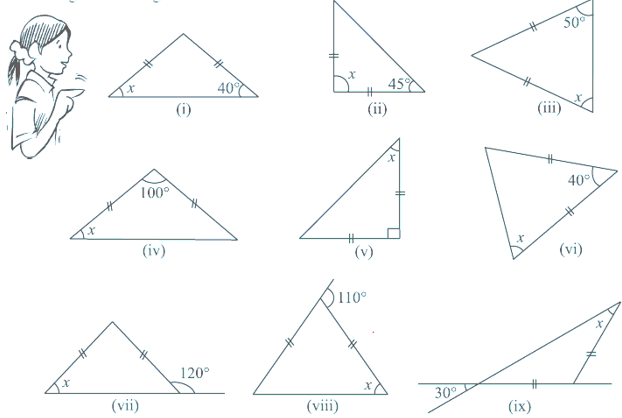i.This is isosceles triangle and x=40°
ii.This is isosceles triangle
$x + 45 + 45=180$
x=90°
iii. This is isosceles triangle and x=50°
iv.This is isosceles triangle
$x + x + 100=180$
x=40°
v. This is isoceles triangle
$x + x + 90=180$
x=45°
vi. This is isosceles triangle
$x + x + 40=180$
x=70°
vi.
This is isosceles triangle
$x + 120=180$
x=60°
vii.
This is isosceles triangle
$2x=110$
x=55°
viii.
This is isosceles triangle
And from vertically opposite angle
x=30°

11.Find angles x and y in each figure.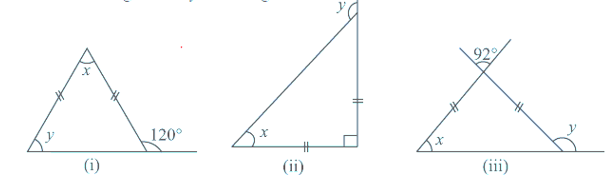a.
This is isosceles triangle
From linear pair
$y + 120 =180$
y=60°
Now
exterior angle=sum of opposite ineterior angles
120=x+60
x=60°
b. This is isosceles right angle triangle
$x + x + 90=180$
x=45°
Now
exterior angle=sum of opposite ineterior angles
y=45 + 90=135°
c. This is isosceles triangle
So ,another angle is also x
Now from vertically opposite angle, third angle =92°
Now from angle property
$x +x +92 =180$
x=44°
Now
exterior angle=sum of opposite ineterior angles
y= 44 + 92 = 136°

### Exercise 6.4

Question 1
Is it possible to have a triangle with the following sides?
(i) 2 cm, 3 cm, 5 cm
(ii) 3 cm, 6 cm, 7 cm
(iii) 6 cm, 3 cm, 2 cm
Solution
In a triangle, the sum of the lengths of either two sides is always greater than the third side.
(i) Given that, the sides of the triangle are 2 cm, 3 cm, 5 cm. It can be observed that,
2 + 3 = 5 cm
However, 5 cm = 5 cm
Hence, this triangle is not possible.
(ii) Given that, the sides of the triangle are 3 cm, 6 cm, 7 cm. Here, 3 + 9 =12 cm > 7 cm
6 + 7 = 13 cm > 3 cm
3 + 7 = 10 cm > 6 cm
Hence, this triangle is possible.
(iii) Given that, the sides of the triangle are 6 cm, 3 cm, 2 cm.
Here, 6 + 3 = 9 cm > 2 cm
However, 3 + 2 = 5 cm < 6 cm
Hence, this triangle is not possible.

Question 2
Take any point O in the interior of a triangle PQR. Is
(i) OP + OQ > PQ?
(ii) OQ + OR > QR?
(iii) OR + OP > RP?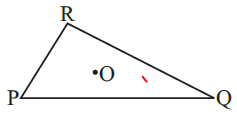Solution
Joining the points the OP, OP and OR
(i) In DOPQ
Sum of two sides > third side
OP + OQ > PQ
(ii) In DOQR
Sum of two sides > third side
OR + OQ > QR
(iii) In DOPR
Sum of two sides > third side
OP + OR > PR
Question 3
AM is a median of a triangle ABC.
Is AB + BC + CA > 2 AM?
(Consider the sides of triangles DABM and DAMC.)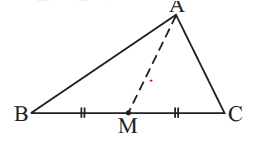Solution
In a triangle, the sum of the lengths of either two sides is always greater than the third
side.
In DABM,
AB + BM > AM (1)
Similarly, in DACM,
AC + CM > AM (ii)
AB + BM + MC + AC > AM + AM
AB + BC + AC > 2AM

Question 4
Is AB + BC + CD + DA > AC + BD?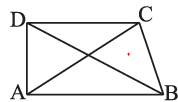Solution
Considering DABC,
AB + BC > CA (i)
In DBCD,
BC + CD > DB (ii)
In DCDA,
CD + DA > AC (iii)
In DDAB,
DA + AB > DB (iv)
Adding equations (I), (//), (iii), and (iv), we obtain
AB + BC + BC + CD + CD + DA + DA + AB > AC + BD + AC + BD
2AB + 2BC + 2CD +2DA > 2AC + 2BD
AB + BC + CD + DA > AC + BD
Question 5
Is AB + BC + CD + DA < 2 (AC + BD)?
Solution
Let O be the point of intersection of diagonals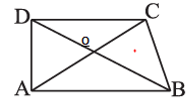In a triangle, the sum of the lengths of either two sides is always greater than the third
side.
In DOAB
OA +OB > AB
In DOBC
OB + OC > BC
In DOCD
OC + OD > CD
OA + OB + OB +OC + OC +OD + OA +OD > AB + BC + CD + DA
2( AC + BD) > AB + BC + CD + DA
Important question
Question 6
The lengths of two sides of a triangle are 12 cm and 15 cm. Between what two measures should the length of the third side fall?
Solution
There are two things for the triangle
(i) sum of the lengths of any two sides of a triangle is greater than the length of the third side.
(ii) difference between the length of any two sides of a triangle is smaller than the length of the third side.
Let x be the third side
So, from first point
12+15 > x
Or x < 27 cm
Now from second point
15-12 < x
Or x > 3
So x lies between 3 cm and 27 cm

### Exercise 6.5

Question 1
PQR. is a triangle right angled at P. If PQ = 10 cm and PR. = 24 cm, find QR
Solution
In DPQR , right angles at P
PQ2 + PR2 = QR2
100 +576 = QR2
QR=26 cm
Question 2
ABC is a triangle, right-angled at C. If AB = 25cm and AC = 7 cm, find BC.
Solution
In DABC , right angles at C
AC2 + BC2 = AB2
BC2 = AB2 -AC2
BC2 = 625 -49 =576
BC=24 cm
Question 3
A 15m long ladder reached a window 12 m high from the ground on placing it against a wall at a distance a. Find the distance of the foot of the ladder from the wall.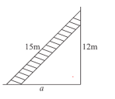Solution
By applying Pythagoras theorem,
(15)2 = (12)2 + a2
225 = 144 + a2
a2 = 225 - 144 = 81
a = 9 m
Question 4
Which of the following can be the sides of a right triangle?
(i) 2.5 cm,6.5 cm, 6 cm.
(ii) 2 cm, 2 cm, 5 cm.
(iii) 1.5 cm, 2cm, 2.5 cm.
In the case of right-angled triangles, identify the right angles.
Solution
i) 2.5cm,6.5cm,6cm
We see that
(2.5)2+62=6.25+36=42.25= (6.5)2
Therefore, the given lengths can be the sides of a right triangle. Also, the angle between the lengths, 2.5 cm and 6 cm is a right angle
ii) 2 cm, 2 cm, 5 cm.
Here 2+ 2= 4 < 5 , So sum of two sides less than third side. So this is not a triangle
iii) 1.5 cm, 2cm, 2.5 cm
We see that
(1.5)2+22=2.25+4=6.25=(2.5)2
Therefore, the given lengths can be the sides of a right triangle. Also, the angle between the lengths, 1.5 cm and 2 cm is a right angle

Question 5
A tree is broken at a height of 5 m from the ground and its top touches the ground at 12 m from the base of the tree. Find the original height of the tree
Solution
Let the tree be broken at point A
Then tree length = AB + AC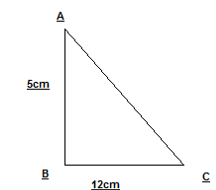In DABC ,right angle at B
AB2 + BC2 = AC2
AC2 = 25 +144 =169
AC=13 m
So original length of tree= 5+13 =18 m
Question 6
Angles Q and R of a DPQR are 25º and 65º.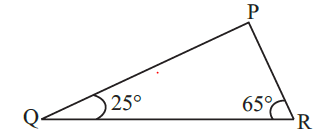Write which of the following is true:
(i) PQ2 + QR2 = RP2
(ii) PQ2 + RP2= QR2
(iii) RP2 + QR2 = PQ2

Solution
From angle property of triangle
Angle P = 180 -25-65=900
So D PQR is right angle at P
So
PQ2 + RP2= QR2
Question 7
Find the perimeter of the rectangle whose length is 40 cm and a diagonal is 41 cm.
Solution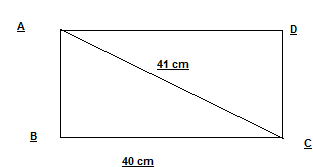Let ABCD is the rectangle as shown above
Now DABC is right angle triangle, So
BC2 + AB2 = AC2
AB2 = 1681 -1600=81
AB=9 cm
So perimeter of rectangle
= 2( AB + BC) = 98 cm
Question 8
The diagonals of a rhombus measure 16 cm and 30 cm. Find its perimeter.
Solution
Let ABCD is the rhombus and the diagonals bisect at right angles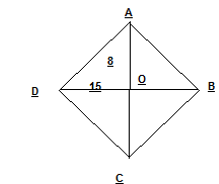$AD^2 = OA^2 + OD^2 = 64 + 225 = 289$
$AD = 17 cm$
So , perimeter of rhombus = 4 X AD= 68 cm

## Similar type Practice questions

(a) classify triangles based on the (i) sides (ii) angles.
(b) What is a median
(c) What can you say about each of the interior opposite angles, when the exterior angle is
(i) a right angle? (ii) an obtuse angle? (iii) an acute angle?
(d) Two angles of a triangle are 45° and 80°. Find the third angle.
(e) One of the angles of a triangle is 60° and the other two angles are equal. Find the measure of each of the equal angles.
(f) The three angles of a triangle are in the ratio 1:2:3. Find all the angles of the triangle.
(g) Can these be sides of the triangle
(i) 2, 4, 7
(ii) 3,4,5
(iii) 10.2 , 5.8 , 4.5
(h) What is the angle sum property of a triangle

Assignments